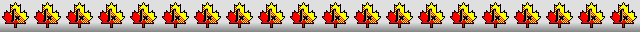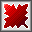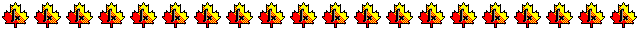Maple worksheets on the derivation of order 6 Runge-Kutta schemesOrder conditions for an order 6 Runge-Kutta scheme - RKcoeff6a.mws

• Order conditions for an order 6 Runge-Kutta scheme and their associated rooted trees.

Derivation of 7 stage, order 6 Runge-Kutta schemes - RKcoeff6b.mws

• Two schemes of John Butcher, Chammud's scheme etc.
See: (1)  "On Runge-Kutta Processes of High Order", by J. C. Butcher,
Journal of the Australian Mathematical Society, Vol. 4, (1964), pages 179 to 194.
(2)  "A three-dimensional family of seven-step Runge-Kutta methods of order 6", by G. M. Chammud (Hammud),
Numerical Methods and programming, 2001, Vol.2, 2001, pages 159-166
(3)  "Optimal first- to sixth-order accurate Runge-Kutta schemes", by E. A. Alshina, E. M. Zaks and N. N. Kalitkin.
Computational Mathematics and Mathematical Physics, Vol. 48, No. 3, pages 395-405.

• Coefficients for the schemes and some properties
RKcoeff6b_1.pdf     RKcoeff6b_2.pdf     RKcoeff6b_3.pdf     RKcoeff6b_4.pdf     RKcoeff6b_5.pdf     RKcoeff6b_6.pdf     RKcoeff6b_7.pdf

Derivation of 8 stage, order 6 Runge-Kutta schemes - RKcoeff6c.mws

• Two schemes of Anton Huta and related schemes.
See: (1)  "Une amélioration de la méthode de Runge-Kutta-Nyström pour la résolution numérique des équations différentielles du premičr ordre", by Anton Huta,
Acta Fac. Nat. Univ. Comenian Math., Vol. 1, pages 201-224 (1956).
(2)  "Contribution ŕ la formule de sixičme ordre dans la méthode de Runge-Kutta-Nyström", by Anton Huta,
Acta Fac.Nat. Univ. Comenian Math., Vol. 2, pages 21-24 (1957).

• Coefficients for the schemes and some properties
RKcoeff6c_1.pdf     RKcoeff6c_2.pdf     RKcoeff6c_3.pdf     RKcoeff6c_4.pdf     RKcoeff6c_5.pdf

Derivation of 7 stage, order 6 Runge-Kutta schemes - RKcoeff6d.mws

• A general stage-order 2 scheme with examples by John Butcher etc.
See: "On Runge-Kutta Processes of High Order", by J. C. Butcher,
Journal of the Australian Mathematical Society, Vol. 4, (1964), pages 179 to 194.

• Coefficients for the schemes and some properties
RKcoeff6d_1.pdf     RKcoeff6d_2.pdf     RKcoeff6d_3.pdf     RKcoeff6d_4.pdf     RKcoeff6d_5.pdf

Derivation of 8 stage, combined order 5 and 6 Runge-Kutta schemes - RKcoeff6e.mws

• Schemes of Prince-Dormand and Jim Verner etc.
See: (1)  "High order embedded Runge-Kutta formulae", by P.J. Prince and J. R. Dormand,
Journal of Computational and Applied  Mathematics, 7 (1981), pp. 67-75.
(2)  A Contrast of a New RK56 pair with DP56, by Jim Verner,
Department of Mathematics. Simon Fraser University, Burnaby, Canada,  2006.

• Coefficients for the schemes and some properties
RKcoeff6e_1.pdf     RKcoeff6e_2.pdf     RKcoeff6e_3.pdf     RKcoeff6e_4.pdf     RKcoeff6e_5.pdf     RKcoeff6e_6.pdf

Derivation of 8 stage, order 6 Runge-Kutta schemes, each with a 9 stage order 5 FSAL embedded scheme - RKcoeff6f.mws

Derivation of 8 stage, order 6 Runge-Kutta schemes, each with a 9 stage order 5 FSAL embedded scheme - RKcoeff6g.mws

• A scheme due to S.N. Papakostas together with similar schemes.
See: "On Phase-Fitted modified Runge-Kutta Pairs of order 6(5)", by Ch. Tsitouras and I. Th. Famelis,
International Conference of Numerical Analysis and Applied Mathematics, Crete, (2006) Extended Abstract.
Note: The scheme described in this paper was originally constructed by S.N. Papakostas.

• Coefficients for the schemes and some properties
RKcoeff6g_1.pdf     RKcoeff6g_2.pdf     RKcoeff6g_3.pdf     RKcoeff6g_4.pdf     RKcoeff6g_5.pdf

Derivation of 8 stage, order 6 Runge-Kutta schemes, each with a 9 stage order 5 FSAL embedded scheme - RKcoeff6h.mws

Derivation of 8 stage, order 6 Runge-Kutta schemes, each with a 9 stage order 5 FSAL embedded scheme - RKcoeff6k.mws

Derivation of 7 stage, order 6 Runge-Kutta schemes, each with an 8th stage to give an embedded order 5 scheme - RKcoeff6m.mws

• Schemes similar to those in the family described by Luther.
See: "An Explicit Sixth Order Runge-Kutta Formula", by H.A.Luther,
Mathematics of Computation, Vol 22, No. 102, April 1968, pages 434-436.

• Coefficients for the schemes and some properties
RKcoeff6m_1.pdf     RKcoeff6m_2.pdf     RKcoeff6m_3.pdf     RKcoeff6m_4.pdf     RKcoeff6m_5.pdf

Derivation of 8 stage, order 6 Runge-Kutta schemes, each with a 9 stage order 5 FSAL embedded scheme - RKcoeff6n.mws

• Stage-order 2 schemes of Papakostas, Tsitouras and Papageorgiou together with similar schemes.
See: "A General Family of Explicit Runge-Kutta Pairs of Order 6(5)", by S. N. Papakostas, Ch. Tsitouras and G. Papageorgiou,
Siam Journal of Numerical Analysis, Volume 33, Number 3, June 1996, pages 917 to 936.

• Coefficients for the schemes and some properties
RKcoeff6n_1.pdf     RKcoeff6n_2.pdf     RKcoeff6n_3.pdf     RKcoeff6n_4.pdf     RKcoeff6n_5.pdf     RKcoeff6n_6.pdf

Derivation of 8 stage, order 6 Runge-Kutta schemes, each with a 9 stage order 5 FSAL embedded scheme - RKcoeff6p.mws

• A stage-order 3 scheme of Lockyer, McCorrigan and Prince together with similar schemes.
See: "Global Error estimation with Runge-Kutta triples", by J.R.Dormand, M.A.Lockyer, N.E.McCorrigan and P.J.Prince,
Computers and Mathematics with Applications, 18 (1989) pages 835-846.

• Coefficients for the schemes and some properties
RKcoeff6p_1.pdf     RKcoeff6p_2.pdf     RKcoeff6p_3.pdf     RKcoeff6p_4.pdf     RKcoeff6p_5.pdf

Derivation of 7 stage, order 6 Runge-Kutta schemes - RKcoeff6q.mws

• Stage-order 3 schemes of Lawson and Tsitouras-Papakostas etc.
See: (1)  "An Order 6 Runge-Kutta Process with an Extended Region of Stability, by J. D. Lawson,
Siam Journal on Numerical Analysis, Vol. 4, No. 4 (Dec. 1967), pages 620 to 625.
(2)  "Cheap Error Estimation for Runge-Kutta methods", by Ch. Tsitouras and S.N. Papakostas,
Siam Journal on Scientific Computing, Vol. 20, Issue 6, Nov 1999.

• Coefficients for the schemes and some properties
RKcoeff6q_1.pdf     RKcoeff6q_2.pdf     RKcoeff6q_3.pdf     RKcoeff6q_4.pdf     RKcoeff6q_5.pdf     RKcoeff6q_6.pdf

Derivation of 7 stage, order 6 Runge-Kutta schemes, each with an 8th stage to give an embedded order 5 scheme - RKcoeff6r.mws

• Two schemes of Tanaka, Kasuga, Yamashita and Yazaki etc.
See: "On the Optimization of Some Seven-stage Sixth-order Runge-Kutta Methods", by M. Tanaka, K. Kasuga, S. Yamashita and H. Yazaki,
Journal of the Information Processing Society of Japan, Vol. 33, No. 8 (1992), pages 993 to 1005.

• Coefficients for the schemes and some properties
RKcoeff6r_1.pdf     RKcoeff6r_2.pdf     RKcoeff6r_3.pdf     RKcoeff6r_4.pdf     RKcoeff6r_5.pdf     RKcoeff6r_6.pdf

Derivation of 8 stage, order 6 Runge-Kutta schemes, each with a 9th stage to give an embedded order 5 scheme - RKcoeff6s.mws

• Six schemes of Tanaka, Kasuga, Yamashita and Yazaki etc.
See: "On the Optimization of Some Eight-stage Sixth-order Explicit Runge-Kutta Method", by M. Tanaka, K. Kasuga, S. Yamashita and H. Yazaki,
Journal of the Information Processing Society of Japan, Vol. 34, No. 1 (1993), pages 62 to 74.

• Coefficients for the schemes and some properties
RKcoeff6s_1.pdf     RKcoeff6s_2.pdf     RKcoeff6s_3.pdf     RKcoeff6s_4.pdf     RKcoeff6s_5.pdf     RKcoeff6s_6.pdf

Derivation of 8 stage, order 6 Runge-Kutta schemes, each with a 9th stage to give an embedded order 5 scheme - RKcoeff6t.mws

Derivation of 7 stage, combined order 6 and 4 Runge-Kutta schemes - RKcoeff6u.mws

• A scheme of El-Mikkawy and Eisa together with a scheme of Tsitouras and S.N. Papakostas etc.
See: (1) "A general four-parameter non-FSAL embedded Runge–Kutta algorithm of orders 6 and 4 in seven stages,"
by M.E.A. El-Mikkawy and M.M.M. Eisa,
Applied Mathematics and Computation, Vol. 143, No. 2,  (2003) pages 259 to 267.
(2)  Cheap Error Estimation for Runge-Kutta methods, by Ch. Tsitouras and S.N. Papakostas,
Siam Journal on Scientific Computing, Vol. 20, Issue 6, Nov 1999.

• Coefficients for the schemes and some properties
RKcoeff6u_1.pdf     RKcoeff6u_2.pdf     RKcoeff6u_3.pdf     RKcoeff6u_4.pdf     RKcoeff6u_5.pdf     RKcoeff6u_6.pdf

Procedures related to the construction of Runge-Kutta schemes - butcher.zip

Procedures for root-finding and determining minimum points  - roots.zip

Procedures for numerical integration  - intg.zip

Top of page

Main index